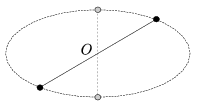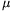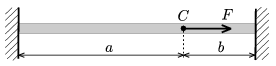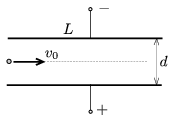Mathematical and Physical Journal
for High Schools
Issued by the MATFUND Foundation
 Already signed up? New to KöMaL?

# KöMaL Problems in Physics, May 2010

Show/hide problems of signs:## Problems with sign 'M'

Deadline expired on June 10, 2010.

M. 306. Measure how the force between two bar magnets, which lie along the same line, depends on the distance between them.

(6 pont)

statistics## Problems with sign 'P'

Deadline expired on June 10, 2010.

P. 4254. A group of amateur astronomers observes a newly discovered binary star. According to their observations the two stars look like a pair of bright points, whose path is the ellipse shown in the figure. Ester, the most enthusiastic member of the group, recently learned Kepler's laws in the high-school. According to her knowledge the celestial bodies really move along elliptical paths, but the attractive centre is not at the symmetry centre O of the ellipse but at one of its foci. She suspects that the two stars revolve about an invisible black hole, which has much greater mass than that of the stars, and which is at the focus of the ellipse. Can Ester be right? How would you explain the observation?(3 pont)

solution (in Hungarian), statistics

P. 4255. What is the direction and the magnitude of the least force which must be exerted on a small object of mass m being at rest at the top of the horizontal table? The coefficients of static and kinetic frictions are equal and.

(4 pont)

solution (in Hungarian), statistics

P. 4256. A rubber cord is stretched out between two walls. At the point of the cord C a force of F along the direction of the cord is exerted.

a) What is the displacement of point C if the cord can be characterized with a spring constant D?

b) At which point of the cord must the force F be exerted when the displacement of C is the greatest?

Data: F=5 N, a=40 cm, b=10 cm,.(4 pont)

solution (in Hungarian), statistics

P. 4257. In a hollow hemisphere a thin ring rolls down without slipping, starting from one endpoint of one of the horizontal diameters. At what speed does it reach the bottom point of the sphere? The radius of the ring is r=2 cm and the radius of the hemisphere is n=4 times as much as that of the ring. The hemisphere is fixed and the plane of its cross section is horizontal.

(4 pont)

solution (in Hungarian), statistics

P. 4258. Three rods, among which any two are perpendicular to each other, are placed onto a rough surface and form a pyramid. The vertex of the pyramid supports a weight of Q. What are the forces exerted by the rods, if they have equal lengths, and if they have different lengths? (The weight of the rods is negligible with respect to the weight Q.)

(5 pont)

solution (in Hungarian), statistics

P. 4259. Why is it possible to turn a somersault after jumping up into the air -- having enough momentum -- and then fall back onto our feet?

(4 pont)

solution (in Hungarian), statistics

P. 4260. An object of mass m can move without friction along the horizontal axis of X. With a thread of length L another object of mass m is connected to it. This object can move only along the vertical axis Y. Initially this object is at the origin of the axes, and the other is at a distance of L on the X axis. Than the objects are left to move. To what speeds will the object accelerates due to the gravitational force? What types of motion do they undergo?

(5 pont)

solution (in Hungarian), statistics

P. 4261. A cylinder of radius 15 cm and of length 50 cm is closed at one end, and 40 cm from the closed end a piston confines a sample of air in standard conditions. The air inside is heated by a heater of power 100 W, which has an efficiency of 80%. How long does it take for the air to push the piston out of the cylinder?

(4 pont)

solution (in Hungarian), statistics

P. 4262. An electron is shot at an initial speed of v0=4.107 m/s into a parallel plate condenser halfway between the plates. The distance between the plates and the length of the plates are unknown, and a constant voltage of U=500 V is applied between the plates. If a uniform magnetic field of induction B=6.25.10-5 Vs/m2 is also applied between the plates, in the appropriate direction, the electron goes through the plates without changing the direction of its motion, at a constant speed.

a) Determine the electric field between the plates of the parallel plate condenser.

b) Find the distance d between the plates.

c) If the magnetic field is ceased the speed of the electron increase by 6% when passes the condenser. What is the length of the plates L?(4 pont)

solution (in Hungarian), statistics

P. 4263. The rate of change of the current in a toroidal coil is 10 A/s. The number of turns of the coil is 2000, its cross section is 2 cm2, and the radius of its central circle is 0.5 m. At what acceleration does a proton or an electron starts to move if it is initially at the centre of the toroidal coil?

Hint: The electric field generated by the varying magnetic field of the toroidal coil is similar to the magnetic field generated by a circular current.

(5 pont)

solution (in Hungarian), statistics